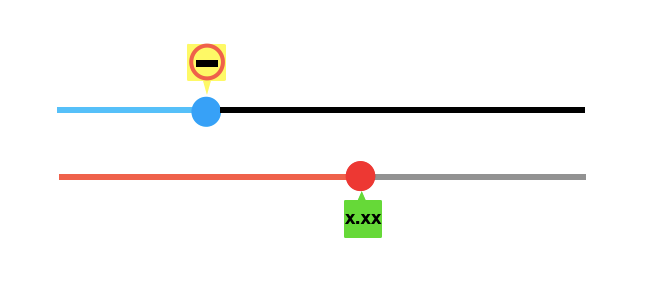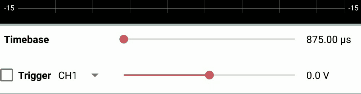# Custom SeekBar in PSLab Android

By default Seekbar in Android only return integer values greater than zero. But there can be some situation where we need the SeekBar to return a float and negative values. To implement trigger functionality in the Oscilloscope activity of PSLab Android app, we require a SeekBar that sets a voltage level that should trigger the capture sequence. Since this voltage value ranges between -16.5 V to 16.5 V, default Seekbar don’t serve the purpose.

The solution is to create a custom SeekBar that returns float values. Let’s understand how to implement it.

Create a FloatSeekBar class which extends AppCompatSeekBar. Create a constructor for the class with Context, AttributeSet and defStyle as parameters. AttributeSet is the set of properties specified in an XML resource file whereas defStyle is default style to apply to this view.

```public class FloatSeekBar extends android.support.v7.widget.AppCompatSeekBar {
private double max = 0.0;
private double min = 0.0;

public FloatSeekBar(Context context, AttributeSet attrs, int defStyle) {
super(context, attrs, defStyle);
applyAttrs(attrs);
}```

Then define setters method which set the max and min values of the SeekBar. This method basically sets the range of the SeekBar.

```public void setters(double a, double b)
{
min = a;
max = b;
}
```

getValue is a method that manipulates current progress of the SeekBar and returns the value. Here the equation used to determine the value is (max – min) * (getProgress() / getMax()) + min.

```public double getValue() {
DecimalFormat df = new DecimalFormat("#.##");
Double value = (max - min) * ((float) getProgress() / (float) getMax()) + min;
value = Double.valueOf(df.format(value));
return value;
}```

setValue method takes the double value, and accordingly set the progress of the SeekBar.

```public void setValue(double value) {
setProgress((int) ((value - min) / (max - min) * getMax()));
}```

This creates a custom SeekBar, it can be used just like a normal SeekBar.

Now, set the range of custom SeekBar between -16.5 and 16.5.

```seekBarTrigger = (FloatSeekBar) v.findViewById(R.id.seekBar_trigger);
seekBarTrigger.setters(-16.5, 16.5);
```

In order to get value of the custom SeekBar call getValue method.

```seekBarTrigger.getValue();
```

In order to follow the entire code for custom SeekBar implementation in PSLab refer FloatSeekBar.java, Attr.xml and TimebaseTrigger.java.

A glimpse of custom SeekBar in PSLab Android.